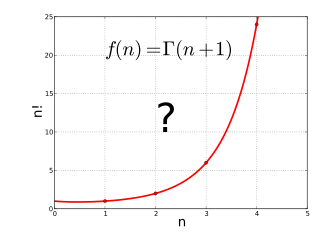How To Create a Code Cracker Game in Python

Code Cracker Tutorial
This tutorial is meant for beginners to intermediate. This tutorial is fairly simple, with some complex concepts to it. In all, this tutorial will get you on your way to learning Python. Without further ado, let's get on with it!

Go here for the output:
Output

If you prefer a video version of this tutorial here it is 😀! Please like and subscribe!
Video

The code on GitHub:
GitHub

The code on Repl.it:
Repl.it

Objective: Generate a random password which is 3 to 5 integers long. Based on the length of the password, we generate the number of lives considering the fact that 'n' integer long password can have n! possible ways of arranging those 'n' numbers. The user has to guess the password based on the computer generated hints provided.Then, click "Python3" in "Popular", or you can search.

Now let's get coding!
First, we need to import the modules needed for our code. So type in:
`import random`
`import time`
Now we can use the files that the creators made in our code! So we do not have to reinvent the wheel.

Let's make our functions to make our program more reusable and easy to understand. We will call these functions later when displaying our hints.

Now, we need to let the player have a certain number of tries before the game ends. This is why we need the factorial function. To make it, type in this code:

``````def factorial(n):
if n == 0:
return True
else:
return n * factorial(n-1)``````

This code will basically puts this equation into code:

n!=n×(n−1)!

or in visuals:Thanks to Wikipedia for the image.

What this code does is that when n, the parameter that the player will input, is 0, the program will stop. If that fails to be true, it subtracts itself until it becomes 0. This is what's called a recursive function (a function which either calls itself or is in a potential cycle of function calls).

Now we have to check if the number is even, so we create a function called even. To do so, we type in the following code:

``````def even(num):
if num % 2 == 0:
return True
else:
return False``````

Quite straightforward, when the value inputted divided by two leaves a remainder of 0, it returns True, telling the program that the number inputted is even.

Now, we need to make a function that counts the number of even numbers. To do so, we type in the following code:

``````def countEven(password):
isEven = 0
if even(int(x)):
isEven += 1
return isEven``````

What this code does is that we use the previous function for them to see if it is even, and it moves the variable isEven up by one. Finally it returns isEven.

We also need to make the function that finds the sum. To do so, we type the following code:

``````def Sum(password):
_sum_ = 0
_sum_ += int(x)
return _sum_``````

In this code, we make the value of the sum in a variable called sum because "sum" is a function in python. And we can't assign a variable the name of a function. This code returns the sum.

The final function we are going to make is the product of all the numbers. This works like Sum(), except with multiplication. This is the code:

``````def product(password):
producty = 1
producty *= int(x)
return producty  ``````

Yay!!! 😀 You made all the functions! Now that we've made them, it's time to call them and display them on the screen. We will use concatenation to join the words together, or in other words, concatenate.

On this line, we choose the length of the code.
`integer = random.randint(3, 5)`
This creates a list of numbers to choose from.
`chars= "1234567890"`
`password = random.sample(chars, integer)`
This determines the lives the player will have.
`lives = factorial(integer)`
This defines the sum.
`_sum_ = Sum(password)`
`print('loading...')`
`time.sleep(random.randint(0, 5))`
Taking out all the weird characters (Delete the next line and put print(password) instead and you'll see what I mean.)
`password = ''.join(password)`
Notifying player the code is generated.
`print("CODE GENARATED")`
Print the hints.

``````print('it is', integer ,'numbers long')
print('the sum is', _sum_)
print('the product is', producty)
print('The number of even numbers is', isEven)
isOdd = integer - isEven
print('The number of odd numbers is', isOdd)
print('you have', lives, 'lives') ``````

Checking if the player has any lives left. If not, it breaks the program, stopping the game.

``````while lives > 0:
print('OMG, you got it ')
break